American Journal of Astronomy and Astrophysics
Volume 2, Issue 5, September 2014, Pages: 47-60

Cosmic redshift in the nonexpanding cellular universe: Velocity-differential theory of cosmic redshift

DSSU Research, 5145 Second Ave., Niagara Falls, Ontario, Canada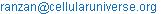Conrad Ranzan. Cosmic Redshift in the Nonexpanding Cellular Universe: Velocity-Differential Theory of Cosmic Redshift. American Journal of Astronomy and Astrophysics. Vol. 2, No. 5, 2014, pp. 47-60

Abstract: A review of the traditional possible causes of cosmic redshift —namely Doppler, expanding vacuum, gravitational, and tired light— is presented along with a discussion of why they failed. A new cosmic redshift mechanism is constructed based on a non-mass, non-energy, space medium (which serves as the luminiferous substrate) and the DSSU cellular cosmology (a remarkably natural problem-free cosmology). The cosmic redshift is shown to be a velocity-differential effect caused by a flow differential of the space medium. Furthermore, the velocity-differential redshift/effect is shown to be part of a much broader unification, since the very mechanism that causes the gravitation effect and sustains the Universe’s gravity-cell structure is also the mechanism that causes the λ elongation manifesting as the cosmic redshift. Agreement with the verifiable portion of the redshift-distance graph (z ≤ 5) is outstanding. The main point is that intrinsic spectral shift occurs with a transit across/through any gravity well (sink). It is caused by the difference in propagation velocity between the axial ends of the photon or wave packet. Which, in turn, is caused by the difference in velocity of the aether flow, the flow differential of the aether, that occurs throughout a gravity well. And here the causal chain is linked to gravity: the change in velocity of the aether flow is what produces the effect of gravitation. The acceleration of the aether flow is the manifestation of gravity.

Keywords: Cosmic Redshift, Photon Propagation, Gravity Cell, Aether, Cellular Cosmology, Redshift distance

1. Background

"It should … be mentioned, as a commentary on the vast fields of mathematics provoked by the linear recession [of galaxies], that its experimental discoverer, Hubble, does not admit that the red-shift is necessarily to be ascribed to the Doppler effect!" –Historian H. T. Pledge, 1939

Cosmic redshift is the term used to describe the nature of any electromagnetic waves (including light waves) that have travelled across some significant cosmic distance —usually many millions of lightyears distance. The electromagnetic waves, quantized as photons, are simply the emissions of the stars within distant star-clusters and galaxies.

The basic observational fact about the cosmic redshift is that the more distant a galaxy’s location, the more its detected light waves have been stretched out —the more the wavelength of the photons have been elongated. The greater a source galaxy’s distance, the greater is the elongation, the more pronounced is the redshift (and the higher is the z-index, the unitless number used to gauge that redshift).

The discovery of the cosmic redshift, historically called the astronomic redshift, is usually accredited to American astronomer Edwin Hubble, but also involved the independent efforts of several other astronomers including Vesto M. Slipher (between 1912 and 1923), the German Carl W. Wirtz (in 1922), and the Swede Knut Lundmark (in 1924). It seems that Vesto Slipher (1875-1969) was the first to measure the spectral shift of an extragalactic object. The theoretical insight of the American cosmologist Howard P. Robertson (in 1928) was also a contributing factor in recognizing the cosmic redshift .

The general concept of the change in the wavelength of light and the causal connection with motion can be traced back to Austrian physicist Johann Christian Doppler (1842); the motion-related changes in wavelength became known as the Doppler effect. The French physicist Hippolyte Fizeau (in 1848) was the first to point out that the shift in spectral lines seen in stars was due to the Doppler effect. (Hence, the effect is sometimes called the Doppler–Fizeau effect.) In 1868, British astronomer William Huggins was the first to determine the velocity of a star moving away from the Earth by this "redshift" method .

1.1. The Possible Causes of Cosmic Redshift

In order to explain the cosmic redshift phenomenon —the phenomenon whereby the measurable redshift increases with the remoteness of the observed galaxy— theorists during the last century came up with four categories of causal explanations, namely:1

Doppler

Expanding space (or space medium)

Gravitational

Tired light

According to the Basic Doppler interpretation:  Galaxies are moving away from us through static space. The greater a galaxy’s distance, the faster it is speeding away and, hence, the larger the redshift. The Doppler interpretation takes its credibility from the fact that a Doppler change in wavelength is a laboratory proven effect. As a practical application, the electromagnetic Doppler effect is key to the operation of speed-measuring radar.

Astronomical objects in motion produce a simple Doppler effect. The light coming from a radiating source moving through space will have an altered wavelength, measured as a blueshift for approaching objects or a redshift for receding objects. The effect serves as a useful tool for astronomers. The problem is that motion through space becomes subject to special relativity and its speed restriction, making it a challenge to explain motion of objects approaching the speed of light as evident from the high redshifts routinely recorded. The fatal flaw in adopting the Basic Doppler interpretation as a cosmological effect, however, is in dealing with the questions: Why are all galaxies, with a few nearby exceptions, moving away from us? Why are we and our Milky Way galaxy located at the center of the universe?

Astronomers and cosmologist soon understood that the "recession speed" associated with the Basic Doppler interpretation was not a motion through space. If it really were the case that all distant galaxies were racing (through static space) away from us, then we would be located at the very center of a remarkable radial pattern of outward bound galaxies —we would occupy a special place in the universe. And that would be a violation of the Copernican principle and its extension, the cosmological principle. That does not happen and cannot be. And so, the Basic Doppler effect was rejected as the mechanism underlying the cosmic redshift.

Expanding space (or space medium) as the cause:  The idea here is that galaxies are more or less stationary within their local region of space in their corner of the universe. There is still a Doppler-like redshift effect; there is still a recession of galaxies. But this time (with the galaxies being locally "stationary") the recession motion is caused by the expansion of intervening space. Under this hypothesis, then, galaxies are moving away from us WITH the expanding vacuum. The greater a galaxy’s distance, the faster it is receding. The argument is that the greater the distance between us and the galaxy, the more intervening space-medium there is; and if that intervening medium is expanding, then it is easy to see how a galaxy’s recession speed —and, hence, cosmic redshift— would be proportional to distance.

Proponents cite the theoretical validation provided by Einstein’s 1917 Equilibrium universe. By virtue of the fact that Einstein’s 1917 universe was supposed to be static but really wasn’t, the model represented the theoretical proof that space (Einstein’s space, the spacetime of general relativity) could not remain static; dynamic expansion, however, was perfectly acceptable. And when space expands, so does the wavelength of any light wave propagating therein.

This connection between space expansion and light-wave elongation only makes sense if Einstein’s space is a luminiferous medium. Although Einstein did not formally abandon his static-universe model until 1932, he readily understood the necessity of a conducting medium for light. His Leyden University lecture, in 1920, made it clear, "according to the general theory of relativity, space is endowed with physical qualities; in this sense, therefore, there exists an [a]ether. According to the general theory of relativity, space without [a]ether is unthinkable; for in such a space there not only would be no propagation of light, but also no possibility of existence for standards of space and time (measuring-rods and clocks), nor therefore any space-time intervals in the physical sense." 

There is no doubt that, in principle and in practice, the expansion of space (or more properly, the expansion of the space medium) as an explanation of the cosmic redshift does work. But it can only be a partial explanation.

The expanding-space-medium interpretation has one major problem —its near universality. In the absence of some countering effect, something to counter the almost universal expansion, this mechanism leads to a rather bizarre but unavoidable configuration. It requires the expansion of the whole universe! The problem with this, hypothesizing a cosmos that expands, is so enormous, so multifaceted, so insurmountable, that it can only lead to a preposterous view of the world. It is simply not possible to build a realistic model of the universe on modes of unrestrained expansion.

Gravitational redshift. In this category there are various mechanisms for the gravitational weakening of light. The earliest of this type probably dates back to Fritz Zwicky’s Gravitational Drag model from the 1920s and 1930s.

According to Einstein’s general relativity, there exists a time dilation effect within a gravitational well, causing a gravitational redshift —sometimes called an Einstein Shift. The theoretical derivation of this effect follows from the Schwarzschild solution of the Einstein equations and gives the redshift associated with a photon travelling in the gravitational field. The following is the predicted (gravitational) redshift that would be detected at the extreme end of a gravity well when measuring a photon that originated at radial distance r from the center of gravity:

Gravitational redshift,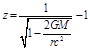(1)

where G is the gravitational constant, M is the mass of the object creating the gravitational field, r is the radial coordinate of the source (which is analogous to the classical distance from the center of the object, but is actually a Schwarzschild coordinate), and c is the speed of light [4,5].

For several decades, the Einstein Shift was merely a theoretical concept, but that changed with the evidence from the famous Pound, Rebka, and Snider experiment. The apparatus was designed to measure the redshift associated with the Earth’s gravitational field. Using the Mössbauer effect Pound and Rebka (in 1959) and Pound and Snider (in 1965) succeeded in measuring the redshift acquired by photons after being emitted from ground level and travelling upward against the Earth’s gravitational pull. The upward distance was only 22.5 meters and the redshift was miniscule, but the results were conclusive. There was a frequency (and wavelength) difference of 2.45 parts in 1015 which represents a gravitational redshift —or fractional loss of energy— of 2.45×10−15. The results agreed within 99.9 percent of the predicted value .

The gravitational redshift can be quite significant for massive, dense, compact stars or star-like objects. But for ordinary stars, as well as extended structures, it is a surprisingly weak effect. In the case of our Sun, when a photon emitted from the surface escapes the Sun’s "gravity well" out to some vast distance it acquires a small redshift of only 2.1 parts per million. That is, the wavelength is stretched by a factor of 2.1×10−6 of the original wavelength as a sole consequence of the gravitational effect .

In the case of a photon that has escaped the gravity well of the Milky Way galaxy, say a photon that had been emitted from the Earth, the acquired redshift would be 0.001 which is still rather small .

What about redshift attributable to the monstrous gravity well of an entire galaxy cluster, say the rich Virgo cluster? A photon emitted from its nominal "surface" at a radius of about 7.5 million lightyears will accumulate an astonishingly small redshift of only 2.5 parts per million —assuming, of course, that the "general relativity" effect is the only one at play. Notice that an entire cluster imparts about the same amount of redshift as one average star! If this seems somewhat strange, keep in mind that Mainstream Physics is still missing an understanding of the causal mechanism of gravity.

Evidently the gravitational mechanism is far, far, too weak to serve as a realistic explanation for the cosmic redshift.

Tired light.  Turning to the "tired light" or "fatigued light" interpretation we find that it is a rather broad category. It includes all manner of mechanisms for distance or time dependent diminishment of the energy of light; but it notably rejects the mechanism of space-medium expansion or contraction. (I mention the latter because it will be shown later that contraction of the luminiferous medium can cause wavelength elongation.) When cosmological redshifts were first discovered, it was Fritz Zwicky who proposed the tired light idea. While usually considered for its historical interest, it is sometimes utilized by nonstandard cosmologies. The idea under this interpretation is that light from distant galaxies might somehow become fatigued on its long journey to us, in some way expending energy during its travels. The loss of energy is reflected in the stretching of the wavelength. Although there was considerable speculation by accredited experts (including George Gamow) intrigued by the tired-light idea seeking explanations by altering the laws of Nature and adjusting the constants of Physics, a convincing cause for the energy loss was, and is, missing. As astrophysicist Edward Wright has stated, "There is no known interaction that can degrade a photon's energy without also changing its momentum, which leads to a blurring of distant objects which is not observed. The Compton shift in particular does not work."

Tired-light hypotheses and the cosmologies that depend on them are not generally considered plausible.

Here is the irresoluble problem: Even if the energy loss mechanism can be made to work, there is a critical feature that simply cannot be explained. There is no way to explain the increased delay between weakened pulses; the increased time intervals between redshifted light pulses. There is no explanation for the elongation of the "gaps" between photons!

Astrophysicists, including G. Burbidge and Halton Arp, while investigating the mystery of the nature of quasars, tried to develop alternative redshift mechanisms but were thwarted by the essential time-stretch feature. It was pointed out in Goldhaber et al "Timescale Stretch Parameterization of Type Ia Supernova B-Band Lightcurves" (ApJ, 558:359–386, 2001) that alternative theories are simply unable to account for timescale stretch observed in the emission profiles of type Ia supernovae.

The tired-light hypotheses/mechanisms cannot explain (i) The elongation of the time interval between light pulses, (ii) nor the duration interval of the bursts of light, such as the duration of supernovae explosions. The more distant such events, the longer they appear to take —the greater their time duration seems to be. No weakened-light concept can deal with this reality.

2. Towards a New Interpretation

Clearly, a new causal explanation of the cosmic redshift is needed, one that avoids the flaws and oversights of the other four categories.

Here are the lessons of the failings detailed in the previous section:

The universe cannot be static. A static cosmos is ruled out by necessity of a dynamic space —that is, the need for a space-medium that can expand and/or contract.

The universe cannot expand. An expanding cosmos is a violation of the philosophical principle that the universe, although it consists of everything there is, is not a thing itself. No action verbal can ever be connected to the Universe. The Universe simply is. Period.

The universe cannot be a single gravitational well. This type of cosmos is ruled out by the theoretical and observational weakness of the gravitational redshift.

The lesson of the tired light hypotheses is that there is no effective substitute to employing space-medium expansion. Expansion seems to be unavoidable. Also, any sort of photon interaction or disturbance mechanisms are to be avoided. It is of great advantage to have a redshift mechanism that does not depend on the photon having to interact with anything other than the universal medium.

For a new interpretation we will turn to a cosmology, which, by an inexplicable error of omission, has never before been considered (at least not before 2002, and not by mainstream theorists). There seems to be no record that a cellularly structured universe has ever been modeled; nothing to be found in the scientific literature of any cosmology theory in which cellularity plays a central role. This seems rather surprising since the real Universe is so obviously cellularly structured. The evidence first emerged from the pioneering efforts of Yakov Boris Zeldovich, Gérard de Vaucouleurs, and Jaan Einasto; and then confirmed by Margaret Geller, John Huchra, A. P. Fairall, and many other astronomers. The evidence is now irrefutable. But the cell structure had always been treated as a more-or-less random phenomenon (influenced by the uncoordinated conflicting "forces" of gravity and Lambda). In response to the overwhelming evidence of cosmic cellular structure from the "dramatic" results of the 2dFGRS, the SDSS and the 2MASS redshift surveys, astrophysicist Rien van de Weygaert and his colleagues suggest that what astronomers observe is a "complex network", the result of "gravitational instability" and "hierarchical gravitational scenarios", just an accidental phenomenon, an arrangement routinely replicated by computer simulations . We turn away from this conventional view. For a new redshift interpretation, it is an intrinsically cellularly structured universe —not merely phenomenologically cellular— that we will turn to. The specific model that holds the greatest potential is the Dynamic Steady State Universe (DSSU). It is essentially a cell theory of cosmology .

2.1. Preliminaries

Be assured that there will be no deviation from the foundation feature of all modern cosmology —the premise that the space medium of the universe expands. This premise and its application to a cellular universe, in accordance with DSSU theory, will serve as our starting point.

The cosmic cell structure is, as one should expect, intimately tied to the mechanism of gravity. And this mechanism of gravity, as has been shown in two recently published papers The Processes of Gravitation and The Dynamic Steady State Universe, is an aether theory of gravity [13,14].  In the context of the cosmic-scale cell structure, the theory essentially states that the space medium expands, flows, and contracts —with the expansion and contraction occurring in separate regions. It is these separate regions that define and sustain the universe’s cellular structure.

The aether itself is like Einstein’s aether in that it is not material —it has no mass and no energy. But unlike Einstein’s aether, which is a continuum, the DSSU aether consists of discrete entities —non-mass, non-energy, entities. One other important characteristic is that, unlike most other theories of gravity, the density of DSSU aether does not vary. Historically, the view has been that gravity was related to the gradient of aether density; and that gravity was some sort of a pressure force imparted by aether; theorists were irresistibly drawn to the notion that the gravity phenomenon was the manifestation of some heterogeneity of the aether. The French physicist Pierre Simon Laplace (1749-1827), for instance, believed that the density of the aether was proportional to the distance from the gravitating body and hypothesized that the force of gravity is generated by the impulse (a pressure) of an aether medium and used the hypothesis to study the motion of planets about the Sun. In the DSSU theory of gravity the count density (spacing density) of the aether entities does not vary. The variation that does occur with the aether —and highly relevant to the cosmic redshift mechanism— is its flow velocity. In fact, the inhomogeneity of this flow of the space medium is the mechanism of Gravity .  The basic aether flow equation is detailed in the Appendix. (The details of the underlying causal mechanism are not important to the present discussion but may be found in  and . But let me just add that the nature of DSSU aether is unique —in a most unexpected way.) We will come back to the inhomogeneous flow shortly. But first we need to understand the nature of the cosmic structure.

The DSSU, as a model of the real universe, is structured as cosmic cells. The cells somehow induce a cosmic redshift on the light travelling through them. Their size is obviously an important factor. So is the nature of the dynamic space medium within. Now, the DSSU theory of gravity predicts that the shape of the cosmic cells is dodecahedral. That is to say, the universe’s void-and-galaxy-cluster network has a correspondence with the interiors, the nodes, and the links of a "packing" of certain polyhedrons. The universe is predicted to be a Euclidean arrangement of rhombic-type dodecahedra.

What interests us is not so much the dodecahedral shape but rather the shape, and particularly the size, of the cells associated with the galaxy clusters located at the nodes of the dodecahedra. If the dodecahedra are the universe’s observable structural cells, then the nodes are the most obvious part of the universe’s gravity cells. Cosmic structural cells are void-centered; cosmic gravity cells are galaxy-cluster-centered. The two, of course, overlap. In order to calculate an average volume occupied by a gravity cell, we do need to know the typical size of the structural cells and also some relevant "solid" geometry.

As for the size, it turns out that the nominal diameter of the structural cells is 350 million lightyears. This diameter is based on the results of a massive 200,000-galaxy survey, which probed within a cosmic volume of about 3 billion lightyears cubed. The recent data, reported in the Monthly Notices of the Royal Astronomical Society ("The WiggleZ Dark Energy Survey: the transition to large-scale cosmic homogeneity"), disprove the hierarchical model in which it is argued, by some theorists, that the entire universe never becomes homogenous and that matter is clustered on ever larger scales, much like one of Mandelbrot's famous fractals. The finding is considered to be extremely significant for cosmology .

In remarkable agreement with the DSSU, the survey essentially revealed that the universe is not hierarchically structured but has a regularity of structure, and that the largest structuring occurs on the scale of 350 million lightyears. Furthermore, since, as the report title claims, "large-scale cosmic homogeneity" begins at this scale, then it follows that the Cosmos is regularly cellular and also that the Universe has a steady state cellular structure. Without some defining steady state aspect there could be no regularity, no "large-scale homogeneity."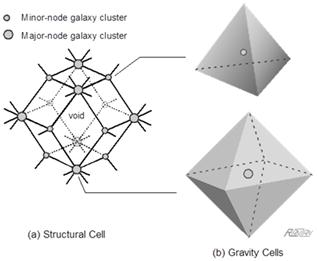Figure 1. The void-and-galaxy-cluster network of the universe is sustained as a close-packing of dodecahedra. Part (a) shows a schematic of an isolated cosmic cell. Its Minor and Major nodes, each of which represents the location of a rich galaxy cluster, are clearly evident. These nodal clusters are the centers of the tetrahedral and octahedral gravity cells. Part (b): The tetrahedron has four vertices; each is the void center of one of four neighboring dodecahedra (which meet at a Minor node). The octahedron has six vertices; each is the void center of one of six neighboring dodecahedra (which meet at a Major node).

Now for the geometry. One of the interesting features of the rhombic-type dodecahedron is that it has two sets of nodes —inner nodes and outer nodes. We will call them Minor and Major nodes. The Minor nodes define the dodecahedron’s inner circumscribing sphere, while the Major nodes define its outer circumscribing sphere. Perhaps the simplest way to define the size of the dodecahedron is to specify its inscribing sphere. Consider a dodecahedron with an inscribed sphere of radius 130 million lightyears (dia. 260 Mly). Then, midway between its inner circumscribing sphere (dia. 320 Mly) and its outer circumscribing sphere (dia. 368 Mly) —almost midway between the two— the diameter is 350 million lightyears. This is the dodecahedron size that best agrees with observations and will serve as the basis for calculating the volumes of the gravity cells.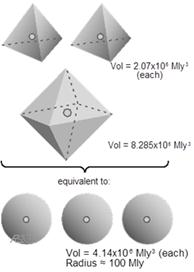Figure 2. Average volume of cosmic gravity cells. For modeling and calculation purposes we conceptually replace the actual tetrahedral and octahedral cells with equivalent spherical cells. (Equivalent in the sense that the number of gravity cells of a region of the universe does not change; the spatial density of the cells, along with their galaxy-cluster nuclei, remains the same.) The close-packing nature of tetrahedra and octahedra demands their presence in the ratio of two to one, respectively.

2.2. Gravity Cells

As pointed out, the void-and-galaxy-cluster network of the universe is sustained as a close-packing of dodecahedra. Now, it so happens that the reciprocal net (also known as the dual network) of the rhombic dodecahedral array consists of both tetrahedra and octahedra .  It means that if all the Minor nodes are regarded as the centers of tetrahedra and all the Major nodes are regarded as the centers of octahedra, then the result is a new close packing —a "no-gaps-space-filling" packing of tetrahedra and octahedra. The nature of the dense packing of dodecahedra means that the shape of the gravity cells must be either tetrahedral or octahedral. Now here is a potential pitfall. When viewed in isolation, as in Fig. 1, it is obvious that there are 8 tetrahedral and 6 octahedral gravity cells surrounding the large cosmic void. (A rhombic dodecahedron has 14 vertices or nodes, unlike the pentagonal dodecahedron which has 20.)  In an extended array, in fact, every such void is surrounded by 8 tetrahedral and 6 octahedral gravity cells. It is easy to be misled into ascribing a ratio of 4 to 3 to the relative abundance of the two shapes. It turns out, however, that the actual ratio of Major to Minor nodes is 2 to 1 and corresponds to the fact that tetrahedra and octahedra can only be close packed in the ratio of 2 to 1. And it is this ratio that is crucial to finding the average volume of the universe’s gravity domains.

First, we need to calculate the volume of the polyhedral gravity cells. We note that each and every "boundary edge" extends from one void-center to an adjacent void-center; and we make use of the geometric fact that the void center-to-center distance is the same as the length of the dodecahedron’s inscribed diameter. Conveniently, all the gravity cells’ "boundary edges" are 260 Mly in length (the same as the inscribed diameter earlier determined based on the observable size of the cosmic dodecahedral cell). Knowing this length allows us to use standard solid geometry formulas. For the tetrahedral gravity cell we have:

Volumetetra gravitycell = 0.1178 (edge length)3 = 0.1178 (260 Mly)3 = 2.07×106 Mly3 .

And for the octahedral gravity cell we have:

Volumeocta gravitycell = 0.4714 (edge length)3 = 0.4714 (260 Mly)3 = 8.285×106 Mly3 .

Notice the large volume difference. This difference helps to explain the size diversity of galaxy clusters.

In order to facilitate the calculation of the cosmic redshift we need to devise a representative gravity cell. Its shape we will simplify as a sphere. Its volume will be based on the weighted average of the volumes and relative populations determined above. The volume is most important; it will ensure that the density of our constructed universe will be the same as the distribution density of clusters in the observed Universe. As shown in Fig. 2, the weighted average of the 2+1 volumes is 4.14×106 Mly3, which is equivalent to a sphere of radius 100 Mly.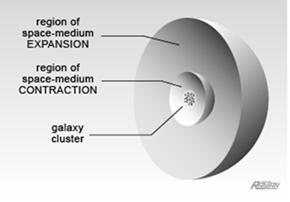Figure 3. Nominal gravity cell (cross-section). This model shows the three essential features of cosmic gravity cells: a large region in which the universal space medium expands, a central region in which the space medium contracts, and a core galaxy cluster. The sphericity is NOT an essential feature. We use the sphere as a convenient representation of the universe's actual tetrahedral and octahedral gravity cells. (Nevertheless, with a radius of 100 million lightyears, it accurately represents the average volume of the domain-of-influence of the host galaxy cluster.)

The sphere is divided into regions of expanding space medium and contracting space medium (Fig. 3). According to DSSU theory, the two dynamics are balanced. The sphere itself neither expands nor contracts. Residing at the sphere’s center is the galaxy cluster. But let me emphasize, this sphere is only a stand-in gravity cell for the universe’s actual gravity cells which are shaped as tetrahedra and octahedra. It serves as a convenient tool to calculate the average redshift across cosmic gravity cells.

The DSSU theory exploits one of the most remarkable symmetries of the Universe —the symmetry between space medium formation (expansion) and space medium annihilation (contraction). The harmonious balance between the two processes sustains the shapes and sizes of the cosmic-scale gravity cells . Of immediate interest is the continuous flow of space medium, or aether, which the expansion and contraction dynamics sustain. We may conceptualize the streaming inward flow of the aether and its velocity gradient as a funnel-like well (Fig. 4). The linear portion of the funnel is associated with homologous expansion, and the curving portion with contractile-gravity-induced accelerated flow. (The latter flow equation is derived in the Appendix.)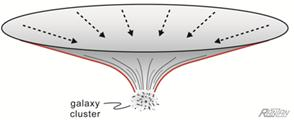Figure 4. Velocity gradient of the space-medium flow occurring within the "nominal" gravity cell is represented as a shallow funnel. Incidentally, it is this flow that sustains the very existence of the matter in the cluster.

Next, we need an exemplary galaxy cluster to place at the heart of our Nominal gravity cell. The nearest rich cluster of galaxies is the Virgo Cluster located between 50 and 60 Mly away from us. It has an estimated mass of 1.2×1015 Solar masses (M) and a radius of about 2.2 Mpc (or 7.2 Mly) .  But note that the Virgo Cluster has several arms that extend beyond the quoted radius.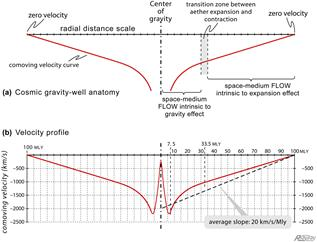Figure 5. Aether inflow graph for (nominal spherical) cosmic gravity cell. Part (a) shows a schematic profile giving key features of the gravity well. Part (b) is the aether velocity profile of the Nominal gravity cell. (Note that at the dead center of the well the aether flow must return to zero.) The average slope of the aether flow profile is 20.0 km/s/Mly.

The anatomy of our gravity cell is detailed, in profile, in Fig. 5a. Notice that at the extreme ends of the schematic well the flow velocity is necessarily zero. The rest of the velocity graph can be constructed as follows. With the aether flow equation (per Appendix),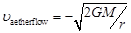(2)

and with mass M = Mcluster = (1.2×1015 M)×(2.0×1030 kg) = 2.4×1045 kg;

and G = 6.67×1030 N m2 kg−2;

and radius r = 7.5 Mly = 70.9×1015 m;

we find that the inflow velocity in the vicinity of the "surface" of the cluster is 2.12×106 m/s, or 2120 km/s, which is plotted as a negative to indicate its radially-inward direction. (See Fig. 5b)

Using simple equation-graphing software we plot (2) as a velocity function of radius r. We do this for the domain [7.5, 40] Mly. A tangent is then drawn to this curve and extended to the gravity-well boundary where the radius equals 100 Mly (and the flow is zero). The point of tangency occurs at the radial distance of 33.5 Mly, where the graph indicates the flow velocity is −1000 km/s. We could, if we wanted to, easily determine the rate-of-expansion constant of the space medium simply by calculating the slope of the tangent. But our interest lies with the average slope of the entire curve, encompassing both expansion and contraction segments. This key information can be extract graphically or numerically.  The average slope is found to be 20.0 km/s/Mly and is crucial for the redshift calculations.

What about the interior of the cluster (the portion between the cluster axis and the cluster "surface")? For this interior part of the profile of Fig. 5b, a simplifying assumption was made regarding the cluster composition. It is assumed that the cluster is completely homogeneous; instead of stars, planets, and galaxies, the cluster is treated as a vast cloud having an equivalent amount of mass. If this cloud-cluster has a linear density gradient, then the aether velocity curve (equation (2) in which mass M includes a linearly variable density) would look very much like the 0 to 7.5 portion of the radial domain of the profile. In reality, however, clusters are enormously clumpy. Superimposed onto the background flow are gravity wells of the cluster’s member galaxies. Since each well requires its own axes, the galaxy wells cannot be represented in Fig. 5. It is surmised that these smaller wells do not materially change the average slope of the main well. If we consider only the main gravity cell, we can be certain that as the inflowing aether penetrates the cluster its speed decreases; and as the aether penetrates to the very center (which invariable is also the center of the dominant galaxy) its speed must ultimately go to zero.

3. Photon Propagation

3.1. Some Essentials

It is a well-understood fact that quanta of light are not point particles; photons are spread-out particles. The longitudinal aspect is measured as the wavelength and defines the photon’s particular energy; the lateral aspect is evident in the phenomenon of polarization.

It is also well-understood that the expansion of the space medium causes an increase in the wavelength of light; and it does so in proportion to the rate of expansion and for as long as the photon propagates within the expanding medium. Virtually every physicist believes this.

Now, if one assumes that medium expansion is responsible for wavelength increase, then one must also accept that medium contraction is responsible for wavelength decrease. If this were unconditionally true we would have a serious problem. Why? If the universe is comprised of balancing regions of expansion and contraction, as indicated in Figures 3, 4, and 5, then one would certainly expect a cancellation effect. There would be practically no cosmic redshift! In a non-expanding cellular universe the redshift, expected under the expansion-contraction mechanism, would be negligible. It all seems very straight forward. However, the above statement is not unconditionally true.

The basic principle is this: When the medium is uniformly expanding, λ always stretches; when the medium is uniformly contracting, λ always shrinks. Remove, or disturb, the uniformity and something remarkable happens. Gravity fields are contraction fields. Within a gravity field, the medium contracts (Einstein called it "contractile" gravity); it contracts non-uniformly. Incidentally, it is this very contraction that conveys the gravitation effect (as detailed in The Processes of Gravitation –The Cause and Mechanism of Gravitation, Journal of Modern Physics and Applications, Vol.2014:3). When a photon within a contractile gravity field travels perpendicular to the medium flow, it contracts —its λ decreases— as might be expected. However, and this is the remarkable part, when a photon, still propagating in a contractile gravity field, travels WITH the medium flow, its λ expands.

Let me make this clear: Within a region where the space medium is contracting, it happens that λ can decrease and also increase. What this means is that environment contraction, if it produces opposite results, cannot be the direct cause. There must be some other factor at play between the medium dynamics (expansion and contraction) on the one hand and the λ response on the other. This "other factor" is able to explain all of the situations/effects discussed above; as well as several other effects of photon propagation. The unifying mechanism is dependent on the photon’s longitudinal aspect and the miniscule difference in velocity that each end of the photon "experiences." The photon is affected by a velocity differential between its leading end and its trailing end.

The velocity differential can be "+" (increasing λ) or "−" (decreasing λ). We will refer to the cumulative effect it has on the photon as the velocity-differential spectral-shift. We may also call it the flow-differential spectral-shift, in recognition of the flow of aether as the cause.

One other essential fact about light propagation: This point has already been assumed, but let me make it explicit. Contrary to what outdated textbooks say, light does require a suitable conducting medium, not a material medium, of course, (not a ponderable medium as Einstein would say) but a medium nevertheless.

Given that the photon is an extended particle, requires a conducting medium, undergoes stretching when the medium expands, and is subject to the flow-differential effect, the following analysis then must be true.

3.2. Outbound Photon

Let us consider a photon emitted from somewhere near the center of the galaxy cluster. Provided the photon encounters no obstacles, its path remains unencumbered, it will emerge from the cluster, pass through the contraction zone, and then, pass through the expansion zone. All the while, the emitted photon, whose original wavelength we will designate as λe, undergoes elongation since there is a propagation velocity difference between the photon’s two ends. (Why this is also true for the inner region of the cluster, in spite of what Fig. 5b seems to indicate, will be explained later.) The propagating photon is shown in Fig. 6. The front and back ends are actually moving apart.

(Relative velocity between ends of photon)
= (vel of front end) − (vel of back end)

= (c + υ1) − (c + υ2) = (υ1 − υ2) > 0 .       (3)

Since υ2 is more positive (that is, higher on the velocity scale) than υ1 (lower on the scale), the expression must be positive. Hence, there is a velocity of separation between the two ends of the photon.

This moving-apart velocity can be expressed as dλ/dt. Furthermore, it is proportional to the wavelength λ itself. In equation form,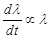.

Introducing a constant/parameter of proportionality we have,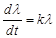(4)

where k is the fractional time-rate-of-change parameter, and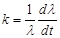(5)

For our representative photon in Fig. 6, λ = (r1r2) and dλ/dt is simply the velocity difference between the photons two ends, which difference, from (3) above, is (υ1 − υ2). Then,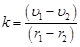(6)

which, by definition, is nothing more than the slope of the dashed velocity line (our linearized flow-function derived earlier).Figure 6.  Photon elongation during outbound part of the journey across cosmic gravity well. The photon is being conducted by a space medium whose speed of inflow decreases by 20.0 km/s (on average) every million lightyears. As a result, the front and back ends of the photon "experience" a flow differential. (The dashed curve is the linearized aether-flow function.)

Our photon is subject to a classic case of uninhibited growth, where the rate of growth (of λ) is proportional to the amount (of length) present.

The wavelength, as a function of time, is found by simple integrating (4):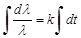(7)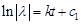,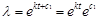,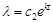.

At time of emission, when t equals zero, λ = λe. Thus, c2 = λe and,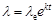(8)

Next, we need the definition of spectral shift,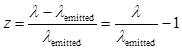(9)

Combining (8) and (9) gives,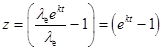(10)

Thus, (ek t – 1) expresses the intrinsic shift acquired by the outbound photon.

Here is how we find the total redshift acquired during the complete outbound trip: We know the value of t. It is just the time it takes for the photon to travel the 100-million-lightyear radius of the gravity cell. And so, t equals 100 Myr. We note that k is 20.0 km/s/Mly and perform a conversion of units (20.0 km/s/Mly = 2.115×10−18 /s  = 6.677×10−5 /Myr).

The resulting outbound (intrinsic) redshift is,

zoutbound = ekt – 1 ≈ 0.00670             (11)

The wavelength elongation and redshift experienced by the photon escaping from a gravity well is, of course, expected. The escape from a gravity well is, after all, associated with a loss of energy.  But what is remarkable, and some may find surprising, is that wavelength elongation also occurs when a photon descends into a gravity well.

3.3. Inbound Photon

We note that the front end of the inbound photon has a greater speed (in the direction of propagation) than does the tail end (Fig. 7). Thus:

(Relative Speed between ends of photon)
= (c + |υ1|) − (c + |υ2|)

= (|υ1| − |υ2|) > 0                   (12)

Hence, there is a relative speed of separation between the two ends of the in-flying photon.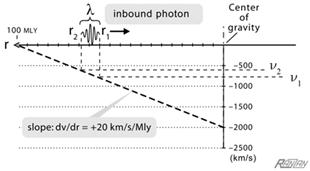Figure 7.  Photon elongation during inbound part of the journey across cosmic gravity well is the result of the front and back ends of the photon "experiencing" a flow differential. (The dashed curve is the linearized aether-flow function.)

The photon undergoes continuous redshifting. That represents the reality for the photon as "measured" in its local space. However, if an observer near the core of the gravity well were to capture this photon in a spectrometer he would not be measuring the full redshift (the intrinsic shift). This is because the observer near the bottom of the cosmic well, say somewhere at the "surface" of galaxy M87, to use our Virgo example, is not really at rest. An observer seemingly "stationary" at a location 60,000 lightyears from the center of M87 would actually be racing through aether at about 2,000 km/s (this being the aether inflow speed at the "surface"). This observer’s motion relative to the aether introduces a significant Doppler shift component.

Let us calculate the intrinsic redshift (independent of any observer). As before the front-and-back separation velocity can be expressed as dλ/dt; and is proportional to the wavelength λ itself. The photon is travelling inward along a radius of the gravity cell and is subject to the equation (which is simply (4) from previous section),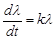,

where λ = (front end) − (back end) = (r1r2); notice that we are using a positive r-axis resulting in a negative value; (r1r2) < 0. And,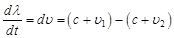= (υ1 − υ2) < 0                 (13)

Then, as before, the fractional time-rate-of-change parameter is,= +20.0 km/s/Mly.    (14)Figure 8. Redshift across Nominal gravity well. Because the front end of the photon is always moving faster than the back end, it undergoes elongation during descent into the gravity well AND during its ascent journey. The photon’s total elongation during its traverse across the cosmic gravity well was calculated to be zGC = 0.0134.

Using (14), with k = +20.0 km/s/Mly and travel time t = 100 Myr, we find the inbound (intrinsic) redshift to be,

zinbound= ekt – 1 ≈ 0.00670             (15)

Thus, the total intrinsic redshift that the photon acquires during a complete transit of the cosmic gravity well is

zGC= 2×0.0067 = 0.0134             (16)

What happens during the photon’s propagation in the interior of the cluster? Photons that penetrate the cloud-cluster, of our thought experiment earlier, without being intercepted or diverted would, according to the velocity-differential principle, become blueshifted. (Recall, the cloud-cluster was simply a temporary assumption we made in connection with Fig. 5b.) But, of course, the interior of the cluster is itself a region of overlapping gravity wells. And herein lies the explanation of why intrinsic redshifting continues within the interior of the galaxy cluster. As photons pass through those successive gravity domains, they continue to acquire velocity-differential redshift.

We now have (as summarized in Fig. 8) all the details of the cosmic gravity cell necessary for testing how well our redshift interpretation agrees with astronomical observations.

4. Testing the Velocity-Differential Interpretation of Cosmic Redshift

Our procedure will be to compare the DSSU’s predicted redshift distances with observational redshift distances corroborated by independent methods (ones not dependent on redshift alone). To make the comparison we will need a redshift-versus-distance expression compatible with DSSU’s cellular structure.

4.1. Derivation of the DSSU’s Redshift-Distance Expression

This simple derivation is based on a dense packing of cosmic gravity cells whose nominal (or average) diameter we designate as DGC.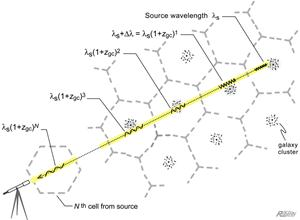Figure 9. Progressive wavelength elongation in a cellular non-expanding universe. Each gravity cell has a nominal diameter of 200 million lightyears and imparts a proportional stretch to the propagating photon. The parameter zGC is the redshift index across a typical cell.

Consider a photon emitted from a galaxy located many cells away. For the photon to reach an Earth detector, it must travel through many cosmic gravity cells (as shown in Fig. 9). The photon starts out with a wavelength (λSource or λS), then as it traverses the first cell, the photon undergoes a proportional elongation. The new wavelength is given by the previous wavelength plus the elongation increment, Δλ. The expression is,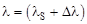, or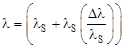.    (17)

But the term (Δλ / λS) is, by definition, the unitless redshift, which, in this case, is attributed to the travel distance across one gravity cell. We make the meaning explicit: we replace the term with the parameter zGC to represent the redshift induced by one cell. With this substitution we can express the photon’s new wavelength, its length after it has passed through the first cell, as,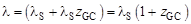(18)

The original wavelength has been transformed by the multiplicative factor of (1+ zGC). As the photon next passes through a similar gravity cell it will again be transformed by a factor of (1+ zGC). So, after passing through the second cell, the new wavelength will be,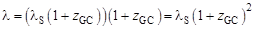(19)

Similarly, after the photon has passed through the third cell, its wavelength will be,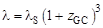(20)

And after passing through N number of cells, the wavelength will be,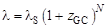(21)

Next, we substitute the latter expression into the definition of the redshift,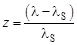,

and obtain the cosmic redshift equation (for the cellular universe) in its basic form,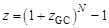(22)

By isolating the cell counter, N, we form an equation of distance expressed solely in terms of redshift. The distance, according to the number of cells between us and the light source, is:(23)

Now, because of the steady-state nature of the processes involved in sustaining the existence of the cells, we expect them to be more or less stable and constitute a Euclidean arrangement. In this arrangement the central galaxy clusters are effectively "stationary points" in a non-expanding universe; and any distance from one cluster to another is Euclidean (regardless of the activity of the intervening space-medium). Thus, the nature of the structure of the DSSU allows for a cosmic distance equation that is remarkably simple.

Distancecosmic= (no. of cells) × (cell diameter) = N × DGC.(24)

Thus, the cellular universe redshift-distance law is: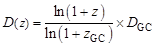(25)

The formula applies specifically to a non-expanding universe having intrinsic-and-stable cellular structure. The expression’s two empirical quantities, the redshift zGC across a single cell and the cell diameter DGC, are both strictly based on observable features. Significantly, the distance function has no arbitrarily adjustable parameters.

4.2. Theory Meets Observational Evidence

The graph of the DSSU redshift-distance expression, referring to (25) with zGC equal to 0.0134 and DGC equal to 200 million lightyears, is illustrated in Fig. 10. It is being compared to an expanding model, the Lambda-cold-dark-matter (ΛCDM) model, for redshift values up to z = 6  [20,21].

The dashed curve is the ΛCDM "theory curve," considered to be the most popular version of the Big Bang. It was designed, with the aid of its several adjustable parameters, to fit the redshift-distance standards that have been established by astronomical observations using methods independent of redshift; the methods involved the use of "standard candles" notably a certain class of supernovae. The dashed ΛCDM curve agrees with observations, for which the margin of error is claimed to be within 5 to 10%. The comparison is revealing. The lesson here, in light of the remarkably close fit of the theory curves and the allowable tolerance, is that if the ΛCDM curve agrees with the data, then unquestionably, so does the DSSU curve!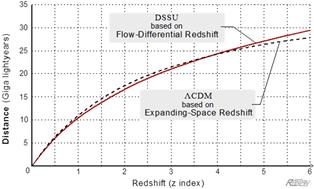Figure 10. Cosmic redshift versus cosmic distance. The velocity-differential interpretation of cosmic redshift fits the narrow observational evidence just as competently as the expanding-space interpretation. The real difference lies with the fit to the broader evidence. The profound difference is that the first is based on the intrinsic cellular structure of the universe and the intrinsic properties of the photon, while the second requires an exploding universe —a wholly unnatural concept. (DSSU model specs: zGC = 0.0134, DGC = 200 Mly; ΛCDM model specs: H0 = 70.0 km/s/Mps, ΩM = 0.27, ΩΛ = 0.73, distance is "now" distance.)

It should be mentioned that expanding universe models make a distinction between the emission distance (the long-ago distance of the source at the time of emission) and the reception distance (the now distance of the source at the time now of deemed detection); the dashed curve in Fig. 10 (as well as in Fig. 11) represents the reception distance. The solid DSSU curve needs no such distinction (since DSSU sources are not receding).

What is truly remarkable is that a non-expanding universe (with no arbitrarily adjustable parameters) fits the data, as evident in the 0-5 portion of the redshift-distance curve for which validity has been independently verified, as well as the expanding-universe model (with its multiple parameters). Keep in mind, in the synthesis of the new interpretation, we did not merely dream up the size of the galaxy cluster; as for the size of the cosmic gravity cell, we did not just pull it out of a hat; and there was nothing arbitrary about how we came up with the flow velocity and velocity differential. All the elements of the flow-differential mechanism are linked to observations as well as being intrinsic to the remarkably simple postulates underlying DSSU theory.

But the DSSU does more than just "fit the data"; with its revolutionary cosmic redshift interpretation, it is profoundly superior, as the next graph will demonstrate. In Fig. 11 the two opposing interpretations of cosmic redshift —the DSSU curve reflecting the flow-differential interpretation, the ΛCDM curve reflecting the evolving-expanding-space interpretation— are extrapolated out to z = 100 .

The graph in Fig. 11 reveals how the expanding-space interpretation leads conventional cosmology to a universe with an artificial boundary. The lower curve is asymptotic at a distance of about 47 Giga lightyears. Extrapolate the redshift-distance graph as far you wish, it will never go far beyond the 47-value line —which represents a visibility limit. The practical effect is simply to compress the depth of field; the more distant the view (of ever higher redshifted objects), the more compressed is the interpretation (of the spacing of the objects). Under the theory that the dashed curve represents, the greater the distance (in terms of its redshift interpretation) the denser the universe appears to be.

This compressed-view problem —this optical illusion seen through the distorted lens of a flawed theory— misleads adherents of Big Bang models into believing that the distant cosmos (and the near cosmos) was much denser in the past.

5. Implications

5.1. Profound Implication

A major implication of the cosmology based on flow-differential redshift, as just discussed, is the absence of any visibility barrier. Distance is a logarithmic function of redshift —a function that rises without limit. The cellular universe is infinite; its Euclidean cellular structure extends to infinity; its dynamic-medium gravity cells repeat forever.

All regions of the universe are either expanding-medium regions or contracting-medium regions in accordance with the DSSU harmony of opposites (with the exception of the various neutral, or zero gravity, Lagrangian points). This Paper has demonstrated how the cosmic redshifting occurs in both kinds of regions. The proof that wavelength elongation occurs in both expanding AND contracting mediums means that the old causal mechanism of cosmic redshift was only half right —and that there is a deeper aspect to the causal mechanism. The implication is that distant galaxies are not receding (ignoring comparatively minor, so called, peculiar motions) and there is no net expansion of the intervening medium. The profound implication is that there is absolutely no need for the Universe to expand!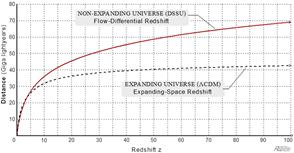Figure 11. Redshift-distance functions extrapolated to redshift z = 100. Different theories make different predictions. The DSSU as a physical Euclidean universe has no spatial limits —the cosmic distance curve increases without limit. The ΛCDM as merely a mathematical construct has an asymptotic distance limit —at approximately 47.2 Giga lighyears. (DSSU specs: zGC = 0.0134, DGC = 200 Mly; ΛCDM specs: H0 = 70.0 km/s/Mps, ΩM = 0.27, ΩΛ = 0.73)

5.2. An Oversight

The research that this Paper represents reveals something else. It has been shown that photon stretching occurs while entering AND exiting the gravity cell. Using the same type of argument the distance between photons also increases. With the aether flow mechanism herein described (the velocity-differential redshift theory), wavelengths dilate, pulse sequences dilate, and "gaps" dilate —and so, the dilation of supernovae light profiles have a simple explanation. Yet, for many years the proponents of the Expanding universe paradigm have been quite emphatically asserting that only a recession-related redshift is able to explain the observed change in the shape of the light curves of supernovae in distant galaxies, which appear to expand exactly by the same factor as the wavelength itself. This dilation phenomenon, they claim, should not be observed if the redshift is not related to the velocity of universal expansion, but instead, has a different physical cause. In other words, all other redshift mechanisms have been ruled out! … The problem is they missed one. What their claim of the exclusive correctness of recession redshift reveals is that the velocity-differential mechanism had never before been examined. It implies an error of omission.

5.3. Lightspeed Independence

A long sought-after goal of astrophysicists has been a formulation of cosmic distance that is independent of the speed of light. Clearly, the new interpretation has succeeded. The intrinsic redshift in conjunction with DSSU’s cosmic gravity wells allows for a redshift-distance equation that does not require c. Furthermore, it does not require an expansion constant; nor does it need any density parameters. The equation is simple and elegant and it works.

5.4. Principle of Intrinsic Spectral Shift

It was noted earlier that intrinsic redshift always occurs where aether expands, but only sometimes where aether contracts. Such inconsistency complicates the design of a principle based on medium dynamics alone. However, a simple and consistent rule is this: Intrinsic redshift always occurs when the absolute speed of the front end of the photon minus the absolute speed of the back end is positive. The principle of intrinsic spectral shift may be expressed as,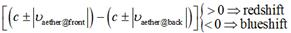(26)

where the "+" is used when the aether flow is in the propagation direction; and "−" when it is opposite to the propagation direction. The principle applies to all situations.

5.5. Applicable to All Gravity Wells

The velocity-differential mechanism is applicable within all gravity wells and is detectable in the vicinity of any gravitating body. It explains the additional redshift that occurs in the "light" from stars during near occultation, when stars pass near the disc of the Sun. Here is a good example. During their observations of the radio source known as Taurus A, Dror S. Sadeh and his colleagues found a significant surge in the redshift of the 21 cm radiation coming from this radio object. They reported that the 21 cm signal suffered a decrease in frequency of 150 hertz (equivalent to a redshift of z = 1.1×10−7) as a consequence of the signal’s passage through the Sun’s gravity well. A total of 20 individual readings were taken on Taurus A while it was located at 1.25 degrees from the Sun on June 15, 1967  They were unable to explain the redshift, noting that it simply cannot be explained by the theory of general relativity, which predicts a shift in frequency of a negligible ±0.16 hertz.

The velocity-differential mechanism also explains the so-called Pioneer-6 anomaly. This is another example of the effect the Solar gravity-well has on photon propagation. It was reported that the 2292 MHz signal from the Pioneer-6 solar-orbit probe was subjected to a pronounced redshift when it passed behind the Sun. And again, there is no satisfactory quantitative general-relativity explanation .

6. Concluding Remarks

Because of their intimate connection with gravity wells, it is worthwhile to note the difference between the conventional gravitational shift and the flow-differential shift.

The conventional shift is treated as the apparent energy change in the photon; a photon emerging from a gravity well loses energy; a photon descending into a gravity well gains energy. Obviously there is a cancellation effect as a photon passes into and then out-of any gravity well. During a cosmic journey in which the photon inevitably encounters countless gravity wells no gravitational shift accumulates —or so the conventional theory predicts. This cancellation is the real reason why Einstein’s gravity shift cannot serve as an explanation of the cosmic redshift.

The gravitational shift is a measure of energy change from the perspective of the observer. Flow-differential shift, on the other hand, is a measure of the intrinsic energy change —a change that is not observer dependent. The differential shift is a measure of the change in λ with respect to the space medium (or, in the case of multiple cosmic gravity cells, with respect to the background frame of the Euclidean universe).

The flow-differential shift is not accurately observable or measurable if you were sitting at some specific spot within the gravity well (unless your aether-referenced motion is negligible or can be compensated). The change in wavelength that the differential shift represents manifests only in the aether frame of reference; an observer must therefore be cognizant of his own local absolute motion and include it in determining the redshift.

There is also a vast difference in the magnitude of the two effects. How weak is the Einstein shift? For the cluster gravity well (shown in Fig. 5) it is 270 times weaker than the flow-differential shift. Hence, in addition to the cancellation problem, the traditional gravitational redshift mechanism is far too weak to be used on the largest scale.

In the search for understanding our World, simple theories that explain a variety of observations in a single unifying framework are most valued. Plate tectonics is an example of such a unifying theory, as it ties together data on minerals and fossils, earthquakes and volcanoes, surface geology and the structure of the Earth’s deep interior. The DSSU aether theory of gravity likewise, albeit on a far wider range of scales, provides a powerful unifying framework. The velocity-differential redshift is just one aspect of a remarkable unification scheme. Within this framework we have an active medium that manifests as contractile gravity as well as Lambda (dark energy) expansion in an unprecedented harmony-of-opposites arrangement . The unifying framework also encompasses cosmic cell structure, galaxy-cluster aspects, galaxy morphology, gravitational lensing, and gravitational collapse (without invoking black hole physics). And, since the medium responsible for gravitation also facilitates the conveyance of photons, the list includes the cosmic redshift. In fact, the cosmic redshift is simply the measurable aspect of the DSSU Gravity-Well Mechanism.

Photon propagation is essentially an excitation-conduction process of aether. Further, since intrinsic spectral shift is determined by what the aether is doing and everything that the aether does is integral to the mechanism of gravity, we would be fully justified in calling the new interpretation the aether-gravity redshift. The flow-differential spectral shift is an aether-gravity shift.

In closing, let me recap and emphasize the main features of the DSSU redshift mechanism:

It is an entirely new concept for the cause of cosmic redshift;

Retains the foundation premise of all modern cosmology, the premise of space-medium expansion;

But does not require whole-universe expansion;

A mechanism that operates for space-medium expansion as well as medium contraction;

A redshift based on the DSSU theory of unified gravity and cosmic cellular structure;

In remarkable agreement with independently established redshift distances.

The velocity-differential interpretation of cosmic redshift, based on a natural cosmology, leads to some truly profound consequences. It makes universal space expansion unnecessary —no need for receding velocities, no need for receding galaxies, and thus, no cosmic Doppler effect. The apparent recession of galaxies is exactly that, apparent —just as Edwin Hubble himself had warned and as historian H. T. Pledge reminded us in the opening quote. If the cosmic redshift is not caused by a Doppler effect, not caused by a recession of galaxies, then the Universe is not expanding. The Universe of the past was not in a dense concentrated state. The Universe did not begin as a big bang.

Appendix

Basic Aether-Inflow Equation

Consider a spherical planet-size mass embedded (at rest) within a stationary aether medium; its mass is represented by M and its radius by R. The inflow-velocity field may be found from Newtonian physics as follows: A small test-mass is resting at some arbitrary distance, r from the center of mass M; it is shown, in Fig. A1, resting just above the sphere’s surface. This small mass, which we designate as m, is "experiencing" a force, in accordance with Newton’s Law of Gravity:

Fgravity = −GMm/r2, where M>>m  and r>R.

From Newton’s 2nd Law of Motion, a force is defined as

F = (mass)×(acceleration),

so that

ma = −GMm/r2.

Although at-rest in the frame of the sphere, the test mass is undergoing acceleration; and whenever there is an acceleration there must be a velocity. This velocity is found by first cancelling the "m" in the above equation, then replacing the acceleration with its definition, a = dυ/dt: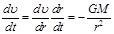,

which (after replacing dr/dt with its identity υ) may be integrated and solved for the velocity.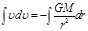,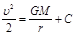, where C = 0 since υ = 0 when r = ∞,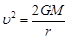.

Note that the test mass is stationary in the sphere reference-frame; it is not accelerating and has no speed with respect to the gravitating body. However, the test mass does have a speed with respect to the aether medium. The υ in the equation represents the relative speed between the test mass and aether.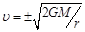,

where G is the gravitational constant and r is the radial distance (from the center of the mass M) to any position of interest, at the surface of M, or external to M. The equation has two solutions. The positive solution expresses the "upward" motion of the test mass through the aether (in the positive radial direction). The negative solution represents the aether flow velocity (in the negative radial direction) streaming past the test mass.

The negative solution represents the speed of inflowing aether at the particular radial location specified by r.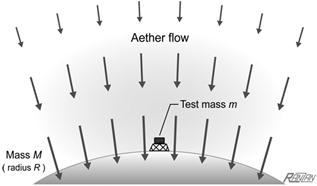Figure A1. Aether streams and accelerates towards and into the large mass. The "stationary" test-mass "experiences" the inflow acceleration as a gravity effect, and "experiences" the inflow speed as a radial component of absolute (aether-referenced) motion according to the formula,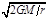References

1. E. R. Harrison, "Chap.11 Redshifts," in Cosmology, the Science of the Universe. (Cambridge University Press, Cambridge, UK, 1981) p231.
2. Wikipedia: http://en.wikipedia.org/wiki/Redshift (Accessed 2014-09-08).
3. A. Einstein, Sidelights on Relativity, Ether and the Theory of Relativity. Translated by G. B. Jeffery and W. Perret, Methuen & Co. London, (1922); republished unabridged and unaltered: Dover, New York, (1983), p 23.
4. E. R. Harrison, "Chap.9 Black holes," in Cosmology, the Science of the Universe. (Cambridge University Press, Cambridge, UK, 1981) p188.
5. Wikipedia: http://en.wikipedia.org/wiki/Redshift (Accessed 2014-09-08).
6. J. A. Wheeler, "Stones in flight," in A Journey Into Gravity and Spacetime. Scientific American Library (New York: W. H. Freeman and Co., 1999) p167.
7. G. O. Abell, "Chap. 35 General relativity," in Exploration of the Universe, 4th Edition. (Saunders College Publishing, New York, NY, 1982) p573-574.
8. E. R. Harrison, "Chap.11 Redshifts," in Cosmology, the Science of the Universe. (Cambridge University Press, Cambridge, UK, 1981) p237.
9. E. L. Wright, "Errors in tired light cosmology," http://www.astro.ucla.edu/~wright/tiredlit.htm (Accessed 2014-09-30).
10. C. Ranzan, "Chap.9 Testing the construction," in Guide to the Construction of the Natural Universe,  (DSSU Research, Niagara Falls, Canada, 2014) p183-192.
11. Rien van de Weygaert, et al, "Geometry and morphology of the cosmic web: Analyzing spatial patterns in the universe," ISVD09 conference (2009).
12. C. Ranzan, "The Dynamic steady state universe," Physics Essays Vol.27, No.2, pp.286-315 (2014).
13. C. Ranzan, "The Processes of gravitation –the cause and mechanism of gravitation," J. Mod. Phys. Appl. Vol.2014:3 (2014).
14. C. Ranzan, "The Dynamic steady state universe," Physics Essays Vol.27, No.2, pp.286-315 (2014).
15. R. T. Cahill,  "Absolute motion and gravitational effects" (Apeiron, Vol.11, No.1, Jan 2004).
16. M. I. Scrimgeour, et al. "The WiggleZ dark energy survey: the transition to large-scale cosmic homogeneity," Monthly Notices of the Royal Astronomical Society, 2012; 425 (1).
17. P. Pearce, Structure in Nature Is a Strategy for Design (The MIT Press, Cambridge, Massachusetts, 1990) p8 & p42.
18. C. Ranzan, "The Dynamic steady state universe," Physics Essays Vol.27, No.2, pp.286-315 (2014).
19. Wikipedia: http://en.wikipedia.org/wiki/Virgo_Cluster (Accessed 2014-03-10).
20. Wikipedia: per http://en.wikipedia.org/wiki/Big_Bang (accessed 2014-08-28).
21. E. Wright, "A cosmology calculator for the world wide web," Publ.Astron.Soc.Pac.118:1711-1715, 2006.Ibid.
22. D. Sadeh, S.H. Knowles, and B.S. Yaplee, "Taurus A experiment," Science, 159, 307 (1968).
23. P. Merat, J.C. Pecker, and J.P. Vigier, Astronomy and Astrophysics, 174, 168 (1974).
24. C. Ranzan, "The Processes of gravitation –the cause and mechanism of gravitation," J. Mod. Phys. Appl. Vol.2014:3 (2014).

Footnotes

 An extensive compilation of cosmological redshift models is included in a recent study by Louis Marmet’s, On the Interpretation of Red-Shifts: A Quantitative Comparison of Red-Shift Mechanisms (2014, July). Marmet gives a quantitative description of the redshift-distance relationship for theoretical mechanisms. For each mechanism a description is given with its properties, limits of applicability, functional relationships and a discussion.

 Contents 1. 1.1. 2. 2.1. 2.2. 3. 3.1. 3.2. 3.3. 4. 4.1. 4.2. 5. 5.1. 5.2. 5.3. 5.4. 5.5. 6.
Article ToolsAbstractPDF(1530K)